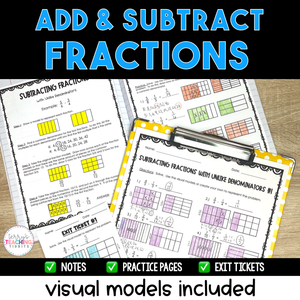# Add and Subtract Fractions - Visual Models Included - Printable

• \$10.00
Unit price per

Use visual models along with the standard algorithm to quickly and effectively teach your students how to add and subtract fractions with like and unlike denominators using this comprehensive resource pack.

Using visual models, students will have a more hands-on approach to “see” what it looks like when adding and subtracting fractions in addition to using the standard algorithm.  It’s great for kinesthetic learners as well as students that may struggle with memorizing algorithms

This resource is complete, comprehensive, and easy to follow with a clear format.  It is low-prep and easy to assign to a sub and contains engaging activities.  If your students are struggling conceptually, this resource is for you!

It is intended for 5th grade, but includes two mini-lessons with unlike denominators in case your students need a review from 4th grade.  It can also be used in a 4th grade math class in which the lessons with unlike denominators can be used for differentiation.

Standards: 5.NF.1, 5.NF.2

This resource is also part of my 5th Grade Math Year-Long Curriculum Bundle.

How Can You Use This?

Introduce each skill with the detailed set of notes and sample problems.  I suggest doing this in a whole group setting and pulling the PDF up on a Smartboard so students can annotate over the models.

Gradually release students to practice independently using the practice pages.   Check for understanding and mastery after teaching each skill using the exit tickets.
When all skills have been taught, provide additional practice and review using the set of task cards, then assess with the final quiz when you feel students are ready.

## What You Get

The Mini-Lessons Included:

• Fraction Notes Pages for Finding the Least Common Multiple, Simplifying Fractions, and Converting Improper Fractions and Mixed Numbers
• Adding Fractions with Like Denominators
• Subtracting Fractions with Like Denominators
• Adding Fractions with Unlike Denominators Using Visual Models
• Subtracting Fractions with Unlike Denominators Using Visual Models
• Adding Fractions with Unlike Denominators Using the Standard Algorithm
• Subtracting Fractions with Unlike Denominators Using the Standard Algorithm

What You’ll Get in EACH of the mini-lessons:

• Detailed Notes
• Sample Problems
• 2 practice pages
• 2 Exit Tickets

You’ll Also Get: